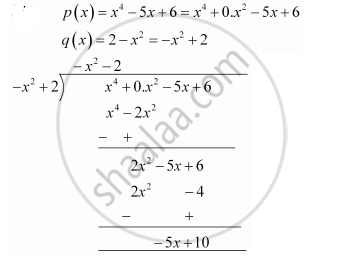Share

Books Shortlist

# Divide the polynomial p(x) by the polynomial g(x) and find the quotient and remainder in each of the following - CBSE Class 10 - Mathematics

ConceptDivision Algorithm for Polynomials

#### Question

Divide the polynomial p(x) by the polynomial g(x) and find the quotient and remainder in each of the following

p(x) = x4 – 5x + 6, g(x) = 2 – x2

#### SolutionQuotient = - x2 - 2

Remainder = - 5x +10

Is there an error in this question or solution?

#### APPEARS IN

NCERT Solution for Mathematics Textbook for Class 10 (2019 to Current)
Chapter 2: Polynomials
Ex.2.30 | Q: 1.3 | Page no. 36

#### Video TutorialsVIEW ALL 

Solution Divide the polynomial p(x) by the polynomial g(x) and find the quotient and remainder in each of the following Concept: Division Algorithm for Polynomials.
S